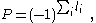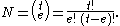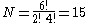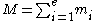xTerm symbolEncyclopedia
In quantum mechanics
Quantum mechanics
Quantum mechanics, also known as quantum physics or quantum theory, is a branch of physics providing a mathematical description of much of the dual particle-like and wave-like behavior and interactions of energy and matter. It departs from classical mechanics primarily at the atomic and subatomic...

, the Russell
Henry Norris Russell
Henry Norris Russell was an American astronomer who, along with Ejnar Hertzsprung, developed the Hertzsprung–Russell diagram . In 1923, working with Frederick Saunders, he developed Russell–Saunders coupling which is also known as LS coupling.-Biography:Russell was born in 1877 in Oyster Bay, New...

-Saunders
term symbol is an abbreviated description of the angular momentum quantum numbers in a multi-electron
Electron
The electron is a subatomic particle with a negative elementary electric charge. It has no known components or substructure; in other words, it is generally thought to be an elementary particle. An electron has a mass that is approximately 1/1836 that of the proton...

atom
Atom
The atom is a basic unit of matter that consists of a dense central nucleus surrounded by a cloud of negatively charged electrons. The atomic nucleus contains a mix of positively charged protons and electrically neutral neutrons...

. It is related with the energy level of a given electron configuration
Electron configuration
In atomic physics and quantum chemistry, electron configuration is the arrangement of electrons of an atom, a molecule, or other physical structure...

. LS coupling is assumed. The ground state term symbol is predicted by Hund's rules.

## Symbol

The term symbol has the form
2S+1LJ

where
S is the total spin quantum number
Spin quantum number
In atomic physics, the spin quantum number is a quantum number that parameterizes the intrinsic angular momentum of a given particle...

. 2S+1 is the spin multiplicity: the maximum number of different possible states of J for a given (L,S) combination.
J is the total angular momentum quantum number.
L is the total orbital quantum number
Azimuthal quantum number
The azimuthal quantum number is a quantum number for an atomic orbital that determines its orbital angular momentum and describes the shape of the orbital...

in spectroscopic notation
Spectroscopic notation
Spectroscopic notation provides various ways to specify atomic ionization states, as well as atomic and molecular orbitals.-Ionization states:Spectroscopists customarily refer to the spectrum arising from a given ionization state of a given element by the element's symbol followed by a Roman numeral...

. The first 17 symbols L are
 L = 0 1 2 3 4 5 6 7 8 9 10 11 12 13 14 15 16 ... S P D F G H I K L M N O Q R T U V (continued alphabetically)There is no official convention for naming angular momentum values greater than 20 (symbol Z). Many papers begin using Greek letters at this point (...). The occasions for which such notation is necessary are few and far between, however.

The nomenclature (S, P, D, F) is derived from the characteristics of the spectroscopic lines corresponding to (s, p, d, f) orbitals: sharp, principal, diffuse, and fundamental, the rest being named in alphabetical order. When used to describe electron states in an atom, the term symbol usually follows the electron configuration
Electron configuration
In atomic physics and quantum chemistry, electron configuration is the arrangement of electrons of an atom, a molecule, or other physical structure...

, e.g., in the case of carbon
Carbon
Carbon is the chemical element with symbol C and atomic number 6. As a member of group 14 on the periodic table, it is nonmetallic and tetravalent—making four electrons available to form covalent chemical bonds...

, the ground state is 1s22s22p2 3P0. The 3 indicates that 2S+1=3 and so S=1, the P is spectroscopic notation for L=1, and 0 is the value of J.

## Others

The term symbol is also used to describe compound systems such as meson
Meson
In particle physics, mesons are subatomic particles composed of one quark and one antiquark, bound together by the strong interaction. Because mesons are composed of sub-particles, they have a physical size, with a radius roughly one femtometer: 10−15 m, which is about the size of a proton...

s or atomic nuclei, or even molecules (see molecular term symbol
Molecular term symbol
In molecular physics, the molecular term symbol is a shorthand expression of the group representation and angular momenta that characterize the state of a molecule, i.e. its electronic quantum state which is an eigenstate of the electronic molecular Hamiltonian. It is the equivalent of the term...

). In that last case, Greek letters are used to designate the (molecular) orbital angular momenta.

For a given electron configuration
• The combination of an S value and an L value is called a term, and has a statistical weight (i.e., number of possible microstates) of (2S+1)(2L+1);
• A combination of S, L and J is called a level. A given level has a statistical weight of (2J+1), which is the number of possible microstates associated with this level in the corresponding term;
• A combination of L, S, J and MJ determines a single state.

As an example, for S = 1, L = 2, there are (2×1+1)(2×2+1) = 15 different microstates corresponding to the 3D term, of which (2×3+1) = 7 belong to the 3D3 (J=3) level. The sum of (2J+1) for all levels in the same term equals (2S+1)(2L+1). In this case, J can be 1, 2, or 3, so 3 + 5 + 7 = 15.

## Term symbol parity

The parity of a term symbol is calculated aswhere li is the orbital quantum number for each electron. In fact, only electrons in odd orbitals contribute to the total parity: an odd number of electrons in odd orbitals (those with an odd l such as in p, f,...) will make an odd term symbol, while an even number of electrons in odd orbitals will make an even term symbol, irrespective of the number of electrons in even orbitals.

When it is odd, the parity of the term symbol is indicated by a superscript letter "o", otherwise it is omitted:
2P has odd parity, but 3P0 has even parity.

Alternatively, parity may be indicated with a subscript letter "g" or "u", standing for gerade (German for 'even') or ungerade ('odd'):
2P½,u for odd parity and 3P0,g for even.

## Ground state term symbol

It is relatively easy to calculate the term symbol for the ground state of an atom. It corresponds with a state with maximal S and L.
Electron configuration
In atomic physics and quantum chemistry, electron configuration is the arrangement of electrons of an atom, a molecule, or other physical structure...

. Full shells and subshells do not contribute to the overall angular momentum
Angular momentum
In physics, angular momentum, moment of momentum, or rotational momentum is a conserved vector quantity that can be used to describe the overall state of a physical system...

• If all shells and subshells are full then the term symbol is 1S0.
2. Distribute the electrons in the available orbital
Atomic orbital
An atomic orbital is a mathematical function that describes the wave-like behavior of either one electron or a pair of electrons in an atom. This function can be used to calculate the probability of finding any electron of an atom in any specific region around the atom's nucleus...

s, following the Pauli exclusion principle
Pauli exclusion principle
The Pauli exclusion principle is the quantum mechanical principle that no two identical fermions may occupy the same quantum state simultaneously. A more rigorous statement is that the total wave function for two identical fermions is anti-symmetric with respect to exchange of the particles...

. First, fill the orbitals with highest ml
Magnetic quantum number
In atomic physics, the magnetic quantum number is the third of a set of quantum numbers which describe the unique quantum state of an electron and is designated by the letter m...

value with one electron each, and assign a maximal ms
Spin quantum number
In atomic physics, the spin quantum number is a quantum number that parameterizes the intrinsic angular momentum of a given particle...

to them (i.e. +½). Once all orbitals in a subshell have one electron, add a second one (following the same order), assigning to them.
3. The overall S is calculated by adding the ms values for each electron. That is the same as multiplying ½ times the number of unpaired electrons. The overall L is calculated by adding the ml values for each electron (so if there are two electrons in the same orbital, add twice that orbital's ml).
4. Calculate J as
• if less than half of the subshell is occupied, take the minimum value ;
• if more than half-filled, take the maximum value ;
• if the subshell is half-filled, then L will be 0, so .

As an example, in the case of fluorine
Fluorine
Fluorine is the chemical element with atomic number 9, represented by the symbol F. It is the lightest element of the halogen column of the periodic table and has a single stable isotope, fluorine-19. At standard pressure and temperature, fluorine is a pale yellow gas composed of diatomic...

, the electronic configuration is 1s22s22p5.

1. Discard the full subshells and keep the 2p5 part. So there are five electrons to place in subshell p .

2. There are three orbitals that can hold up to . The first three electrons can take but the Pauli exclusion principle forces the next two to have because they go to already occupied orbitals.
 ml +1 0 −1 ms: ↑↓ ↑↓ ↑

3. ; and , which is "P" in spectroscopic notation.

4. As fluorine 2p subshell is more than half filled, . Its ground state term symbol is then .

## Term symbols for an electron configuration

To calculate all possible term symbols for a given electron configuration
Electron configuration
In atomic physics and quantum chemistry, electron configuration is the arrangement of electrons of an atom, a molecule, or other physical structure...

the process is a bit longer.
• First, calculate the total number of possible microstates N for a given electron configuration. As before, we discard the filled (sub)shells, and keep only the partially-filled ones. For a given orbital quantum number l the total number of electrons that can be fitted is . If there are e electrons in a given subshell, the number of possible microstates isAs an example, lets take the carbon
Carbon
Carbon is the chemical element with symbol C and atomic number 6. As a member of group 14 on the periodic table, it is nonmetallic and tetravalent—making four electrons available to form covalent chemical bonds...

electron structure: 1s22s22p2. After removing full subshells, there are 2 electrons in a p-level , so we havedifferent microstates.

• Second, draw all possible microstates. Calculate ML and MS for each microstate, withwhere mi is either ml or ms for the i-th electron, and M represents the resulting ML or MS respectively:  ml   +1 0 −1 ML all up ↑ ↑ 1 1 ↑ ↑ 0 1 ↑ ↑ −1 1 all down ↓ ↓ 1 −1 ↓ ↓ 0 −1 ↓ ↓ −1 −1 one up one down ↑↓ 2 0 ↑ ↓ 1 0 ↑ ↓ 0 0 ↓ ↑ 1 0 ↑↓ 0 0 ↑ ↓ −1 0 ↓ ↑ 0 0 ↓ ↑ −1 0 ↑↓ −2 0

• Third, count the number of microstates for each MLMS possible combination  MS   +1 1 1 2 1 1 3 1 1 2 1 1

• Fourth, extract smaller tables representing each possible term. Each table will have the size (2L+1) by (2S+1), and will contain only "1"s as entries. The first table extracted corresponds to ML ranging from −2 to +2 (so ), with a single value for MS (implying ). This corresponds to a 1D term. The remaining table is 3×3. Then we extract a second table, removing the entries for ML and MS both ranging from −1 to +1 (and so , a 3P term). The remaining table is a 1×1 table, with , i.e., a 1S term.  Ms cellspacing="0"> 1 1 1 1 1
cellspacing="0">

S=1, L=1, J=2,1,0

3P2, 3P1, 3P0   Ms   +1 0 −1 Ml +1 1 1 1 0 1 1 1 −1 1 1 1 cellspacing="0">

S=0, L=0, J=0

1S0   Ms   0 Ml 0 1

• Fifth, applying Hund's rules, deduce which is the ground state (or the lowest state for the configuration of interest.) Hund's rules should not be used to predict the order of states other than the lowest for a given configuration. (See examples at Hund's rules#Excited states.)

### Alternative method using group theory

For configurations with at most two electrons (or holes) per subshell, an alternative and much quicker method of arriving at the same result can be obtained from group theory
Group theory
In mathematics and abstract algebra, group theory studies the algebraic structures known as groups.The concept of a group is central to abstract algebra: other well-known algebraic structures, such as rings, fields, and vector spaces can all be seen as groups endowed with additional operations and...

. The configuration 2p2 has the symmetry of the following direct product in the full rotation group:
Γ(1) × Γ(1) = Γ(0) + [Γ(1)] + Γ(2),

which, using the familiar labels , and , can be written as
P × P = S + [P] + D.

The square brackets enclose the anti-symmetric square. Hence the 2p2 configuration has components with the following symmetries:
S + D (from the symmetric square and hence having symmetric spatial wavefunctions);
P (from the anti-symmetric square and hence having an anti-symmetric spatial wavefunction).

The Pauli principle and the requirement for electrons to be described by anti-symmetric wavefunctions imply that only the following combinations of spatial and spin symmetry are allowed:
1S + 1D (spatially symmetric, spin anti-symmetric)
3P (spatially anti-symmetric, spin symmetric).

Then one can move to step five in the procedure above, applying Hund's rules.

The group theory method can be carried out for other such configurations, like 3d2, using the general formula
Γ(j) × Γ(j) = Γ(2j) + Γ(2j-2) + ... + Γ(0) + [Γ(2j-1) + ... + Γ(1)].

The symmetric square will give rise to singlets (such as 1S, 1D, & 1G), while the anti-symmetric square gives rise to triplets (such as 3P & 3F).

More generally, one can use
Γ(j) × Γ(k) = Γ(j+k) + Γ(j+k-1) + ... + Γ(|j-k|)

where, since the product is not a square, it is not split into symmetric and anti-symmetric parts. Where two electrons come from inequivalent orbitals, both a singlet and a triplet are allowed in each case.